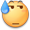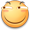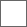• 21回复贴，共1

### 论没🐎ssive的滑稽数值系统与奇异の插件命名疯狂抽楼我佛辣首先我们先看一下面板给的数据：28851*(1+0.83)*(1+0.7)=89755.416

28851*1.83*1.7*(1+0.5)=134633.1915

28851*(1+0.83+0.5)*(1+0.7)=114278.811！28851*(1+0.83+0.005)*(1+0.7)=90000.6945！M组司马干货贴 顶赞

（我也想不通他们成天搞出.5%这种芝麻大的数值是为了个啥，可能是因为这很cooooool